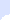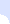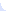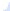# Reseach Projects- Inverse Perturbation for Optimal Intervention in Gene Regulatory NetworksHome Resume Research Projects Publications Teaching Students# Inverse Perturbation for Optimal Intervention in Gene Regulatory Networks

Motivation:
Analysis and intervention in the dynamics of gene regulatory networks is at the heart of emerging efforts in the development of modern treatment of numerous ailments including cancer. The ultimate goal is to develop methods to intervene in the function of living organisms in order to drive cells away from a malignant state into a benign form. A serious limitation of much of the previous work in cancer network analysis is the use of external control, which requires intervention at each time step, for an indefinite time interval. This is in sharp contrast to the proposed approach, which relies on the solution of an inverse perturbation problem to introduce a one-time intervention in the structure of regulatory networks. This isolated intervention transforms the attractor set of the dynamic system to a unique attractor characterized by the desired steady-state distribution.

Results:
We formulate the optimal intervention problem in gene regulatory networks as a minimal-perturbation of the network in order to force it to converge to a desired-steady-state distribution of gene regulation. We cast optimal intervention in gene regulation as a convex optimization problem, thus providing a globally optimal solution which can be efficiently computed for networks with thousands of nodes. The criteria adopted for optimality is chosen to minimize potential adverse effects as a consequence of the intervention strategy. We consider a perturbation that minimizes (i) the overall energy of change between the original and controlled networks and (ii) the time needed to reach the desired steady-state of gene regulation. Furthermore, we show that there is an inherent tradeoff between minimizing the energy of the perturbation and the convergence rate to the desired distribution. We apply the proposed control to the Human melanoma gene regulatory network.

MATLAB & MATHEMATICA Code:

P_matrix.xls

CODE: The MATLAB code uses CVX, a package for specifying and solving convex programs. One needs to download and install the CVX package before running the MATLAB program

Min_energy_perturbation.m with output the minimal-perturbation energy matrix.xls

log file.txt

The code is also displayed below:
0001   %% %%%%%%%%%%%%%%%%%%%%%%%%%%%%%%%%%%%%%%%%%%%%%%%%%%%%%%%
0002   % The program "Min_energy_perturbation.m" reads a square matrix, the
0003   % probability transition matrix of the network, "P_matrix.xls" in excel format and returns the outputs "C", "nu",
0004   % "gamma", and "mu" defined as follows:
0005   % C: the minimal energy perturbation matrix, which forces the network to
0006   % transition from its initial steady-state to the desired steady-state pid.
0007   % nu: the Lagrange multiplier associated with the equality: pid'*(P+C) ==
0008   % pid'
0009   % gamma: the Lagrange multiplier associated with the equality C*OneVec ==
0010   % ZeroVec
0011   % mu: the Lagrange multiplier associated with the inequality P+C >= 0.
0012   %
0013   % This code uses the CVX software. For installation instructions, please
0015   %% %%%%%%%%%%%%%%%%%%%%%%%%%%%%%%%%%%%%%%%%%%%%%%%%%%%%%%%%%
0016
0017
0018   %% Read the probability transition matrix "P_matrix.xls"
0020
0021   %% Global variable definition
0022   N = size(P,1);
0023   OneVec = ones(N,1);
0024   ZeroVec = zeros(N,1);
0025
0026   %% Define the desired steady-state \pi_d
0027   pid1 = 0.015525 * ones(N/2,1);
0028   pid2 = 10^(-4) * ones(N/2,1);
0029   pid = [pid1;pid2];
0030
0031   %% The optimization algorithm using the CVX software
0032   cvx_begin
0033       variable C(N,N);
0034       dual variable nu;
0035       dual variable gamma;
0036       dual variable mu;
0037
0038       minimize( norm(C) );
0039       subject to
0040       nu: pid'*(P+C) == pid';
0041       gamma: C*OneVec == ZeroVec;
0042       mu: P+C >= 0;
0043   cvx_end

plot_Norm_C.m

plot_prop5.m